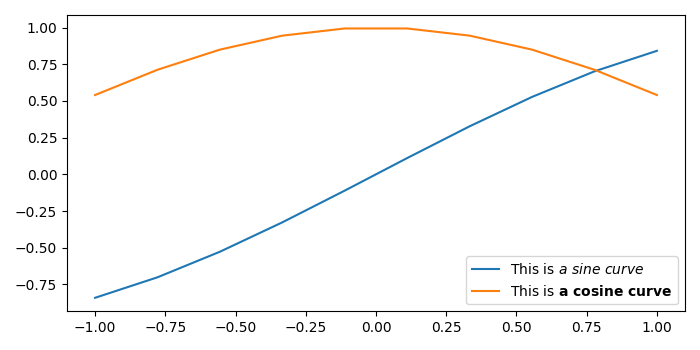# Styling a part of label in legend in Matplotlib

To style a part of label in legend, we can take the following steps −

• Create data point for using numpy.

• Plot a sine curve using np.sin(x) with a text label.

• Plot a cosine curve using np.cos(x) with a text label.

• To place the legend on the plot, use legend() method.

• To display the figure, use show() method.

## Example

import numpy as np
from matplotlib import pyplot as plt
plt.rcParams["figure.figsize"] = [7.00, 3.50]
plt.rcParams["figure.autolayout"] = True
x = np.linspace(-1, 1, 10)
plt.plot(x, np.sin(x), label="This is $\it{a\ sine\ curve}$")
plt.plot(x, np.cos(x), label="This is $\bf{a\ cosine\ curve}$")
plt.legend(loc='lower right')
plt.show()

## Output